Home    |    Teacher    |    Parents    |    Glossary    |    About UsIn a fraction, the denominator tells us how many parts the whole is divided into, and the numerator tells us how many of those parts we're dealing with. To review the parts of a fraction, move your mouse cursor over the fraction below.

Here are some other important fraction terms to review:

Proper fraction: numerator is less than the denominator.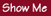Improper fraction: numerator is greater than or equal to denominator.Mixed number: whole number and a fraction.Equivalent fractions: fractions that represent the same
number.Reciprocal: the multiplicative inverse of a number. For a fraction, it's obtained by "turning the fraction over".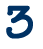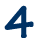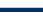Homework Help | Pre-Algebra | FractionsEmail this page to a friendSearch·  Definitions·  Reducing fractions·  Adding and subtract-     ing fractions·  Multiplying fractions·  Dividing fractions·  Adding and subtract-     ing mixed numbers·  Multiplying     mixed numbers·  Dividing     mixed numbers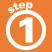First Glance In Depth Examples WorkoutFraction definitions